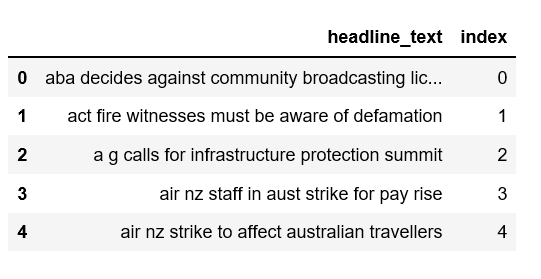# Topic Modeling and Latent Dirichlet Allocation (LDA) in PythonPhoto Credit: Pixabay

Topic modeling is a type of statistical modeling for discovering the abstract “topics” that occur in a collection of documents. Latent Dirichlet Allocation (LDA) is an example of topic model and is used to classify text in a document to a particular topic. It builds a topic per document model and words per topic model, modeled as Dirichlet distributions.

Here we are going to apply LDA to a set of documents and split them into topics. Let’s get started!

# The Data

The data set we’ll use is a list of over one million news headlines published over a period of 15 years and can be downloaded from Kaggle.

```import pandas as pddata = pd.read_csv('abcnews-date-text.csv', error_bad_lines=False);
data_text['index'] = data_text.index
documents = data_text
```

Take a peek of the data.

```print(len(documents))
print(documents[:5])```# Data Pre-processing

We will perform the following steps:

• Tokenization: Split the text into sentences and the sentences into words. Lowercase the words and remove punctuation.
• Words that have fewer than 3 characters are removed.
• All stopwords are removed.
• Words are lemmatized — words in third person are changed to first person and verbs in past and future tenses are changed into present.
• Words are stemmed — words are reduced to their root form.

```import gensim
from gensim.utils import simple_preprocess
from gensim.parsing.preprocessing import STOPWORDS
from nltk.stem import WordNetLemmatizer, SnowballStemmer
from nltk.stem.porter import *
import numpy as np
np.random.seed(2018)import nltk

True

Write a function to perform lemmatize and stem preprocessing steps on the data set.

```def lemmatize_stemming(text):
return stemmer.stem(WordNetLemmatizer().lemmatize(text, pos='v'))def preprocess(text):
result = []
for token in gensim.utils.simple_preprocess(text):
if token not in gensim.parsing.preprocessing.STOPWORDS and len(token) > 3:
result.append(lemmatize_stemming(token))
return result```

Select a document to preview after preprocessing.

```doc_sample = documents[documents['index'] == 4310].valuesprint('original document: ')
words = []
for word in doc_sample.split(' '):
words.append(word)
print(words)
print('\n\n tokenized and lemmatized document: ')
print(preprocess(doc_sample))```

original document:

[‘rain’, ‘helps’, ‘dampen’, ‘bushfires’]

tokenized and lemmatized document:

[‘rain’, ‘help’, ‘dampen’, ‘bushfir’]

It worked!

Preprocess the headline text, saving the results as ‘processed_docs’

processed_docs[:10]Figure 2

# Bag of Words on the Data set

Create a dictionary from ‘processed_docs’ containing the number of times a word appears in the training set.

```dictionary = gensim.corpora.Dictionary(processed_docs)count = 0
for k, v in dictionary.iteritems():
print(k, v)
count += 1
if count > 10:
break```

1 communiti

2 decid

3 licenc

4 awar

5 defam

6 wit

7 call

8 infrastructur

9 protect

10 summit

Gensim filter_extremes

Filter out tokens that appear in

• less than 15 documents (absolute number) or
• more than 0.5 documents (fraction of total corpus size, not absolute number).
• after the above two steps, keep only the first 100000 most frequent tokens.
`dictionary.filter_extremes(no_below=15, no_above=0.5, keep_n=100000)`

Gensim doc2bow

For each document we create a dictionary reporting how many
words and how many times those words appear. Save this to ‘bow_corpus’, then check our selected document earlier.

```bow_corpus = [dictionary.doc2bow(doc) for doc in processed_docs]
bow_corpus```

[(76, 1), (112, 1), (483, 1), (3998, 1)]

Preview Bag Of Words for our sample preprocessed document.

```bow_doc_4310 = bow_corpusfor i in range(len(bow_doc_4310)):
print("Word {} (\"{}\") appears {} time.".format(bow_doc_4310[i],
dictionary[bow_doc_4310[i]],
bow_doc_4310[i]))```

Word 76 (“bushfir”) appears 1 time.

Word 112 (“help”) appears 1 time.

Word 483 (“rain”) appears 1 time.

Word 3998 (“dampen”) appears 1 time.

# TF-IDF

Create tf-idf model object using models.TfidfModel on ‘bow_corpus’ and save it to ‘tfidf’, then apply transformation to the entire corpus and call it ‘corpus_tfidf’. Finally we preview TF-IDF scores for our first document.

```from gensim import corpora, modelstfidf = models.TfidfModel(bow_corpus)
corpus_tfidf = tfidf[bow_corpus]from pprint import pprintfor doc in corpus_tfidf:
pprint(doc)
break```

[(0, 0.5907943557842693),

(1, 0.3900924708457926),

(2, 0.49514546614015836),

(3, 0.5036078441840635)]

# Running LDA using Bag of Words

Train our lda model using gensim.models.LdaMulticore and save it to ‘lda_model’

`lda_model = gensim.models.LdaMulticore(bow_corpus, num_topics=10, id2word=dictionary, passes=2, workers=2)`

For each topic, we will explore the words occuring in that topic and its relative weight.

```for idx, topic in lda_model.print_topics(-1):
print('Topic: {} \nWords: {}'.format(idx, topic))```

Can you distinguish different topics using the words in each topic and their corresponding weights?

Can you distinguish different topics using the words in each topic and their corresponding weights?

# Running LDA using TF-IDF

```lda_model_tfidf = gensim.models.LdaMulticore(corpus_tfidf, num_topics=10, id2word=dictionary, passes=2, workers=4)for idx, topic in lda_model_tfidf.print_topics(-1):
print('Topic: {} Word: {}'.format(idx, topic))```

Again, can you distinguish different topics using the words in each topic and their corresponding weights?

# Performance evaluation by classifying sample document using LDA Bag of Words model

We will check where our test document would be classified.

`processed_docs`

[‘rain’, ‘help’, ‘dampen’, ‘bushfir’]

```for index, score in sorted(lda_model[bow_corpus], key=lambda tup: -1*tup):
print("\nScore: {}\t \nTopic: {}".format(score, lda_model.print_topic(index, 10)))```

Our test document has the highest probability to be part of the topic that our model assigned, which is the accurate classification.

# Performance evaluation by classifying sample document using LDA TF-IDF model.

```for index, score in sorted(lda_model_tfidf[bow_corpus], key=lambda tup: -1*tup):
print("\nScore: {}\t \nTopic: {}".format(score, lda_model_tfidf.print_topic(index, 10)))```

Our test document has the highest probability to be part of the topic that our model assigned, which is the accurate classification.

# Testing model on unseen document

```unseen_document = 'How a Pentagon deal became an identity crisis for Google'
bow_vector = dictionary.doc2bow(preprocess(unseen_document))for index, score in sorted(lda_model[bow_vector], key=lambda tup: -1*tup):
print("Score: {}\t Topic: {}".format(score, lda_model.print_topic(index, 5)))```